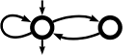Awali Another Weighted Automata library
Standard

Contains all functions related to standard automata. More...

## Functions

automaton_t awali::dyn::left_mult (automaton_t aut, weight_t w, options_t opts={})
Produces an automaton that associates with every word the weight (`w` * x), where x is the weight associated with this word in `aut`. More...

automaton_t awali::dyn::right_mult (automaton_t aut, weight_t w, options_t opts={})
Produces an automaton that associates with every word the weight (x * `w`), where x is the weight associated with this word in `aut`. More...

automaton_t awali::dyn::internal::standard (ratexp_t exp)

## Detailed Description

Contains all functions related to standard automata.

## ◆ left_mult()

 automaton_t awali::dyn::left_mult ( automaton_t aut, weight_t w, options_t opts = `{}` )

Produces an automaton that associates with every word the weight (`w` * x), where x is the weight associated with this word in `aut`.

This function has two different behaviours depending on whether `aut` is standard or not:

• if `aut` is standard : multiplies the weight of each outgoing transition of the initial state by `w`; (in this case the returned automaton is standard)
• if `aut` is not standard : multiplies every initial weight by `w` on the left.

The following options may be given in `opts:` KEEP_HISTORY, IN_PLACE. (Other options are ignored)

If IN_PLACE is `true`, this operation operation is done directly on `aut`. In this case, KEEP_HISTORY is ignored.

If IN_PLACE is `false, this operation is done on a copy of `aut`.

Parameters
 aut automaton (unchanged) w weight by which the initial weights are multiplied opts a set of options
Returns
a copy of `aut` in which the transition weights have been multiplied by `w`

## ◆ right_mult()

 automaton_t awali::dyn::right_mult ( automaton_t aut, weight_t w, options_t opts = `{}` )

Produces an automaton that associates with every word the weight (x * `w`), where x is the weight associated with this word in `aut`.

Consists in multiplying every final weight by `w` on the right.

The following options may be given in `opts:` KEEP_HISTORY, IN_PLACE. (Other options are ignored)

If IN_PLACE is `true`, this operation operation is done directly on `aut`. In this case, KEEP_HISTORY is ignored.

If IN_PLACE is `false`, this operation is done on a copy of `aut`.

Parameters
 aut automaton (unchanged) w weight by which the final are multiplied opts a set of options
Returns
a copy of `aut` in which the transition weights have been multiplied by `w`

## ◆ standard()

 automaton_t awali::dyn::internal::standard ( ratexp_t exp )
Deprecated:
Use exp_to_aut`(exp,{EXP_TO_AUT_ALGO=STANDARD})` instead.